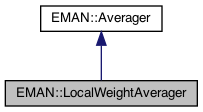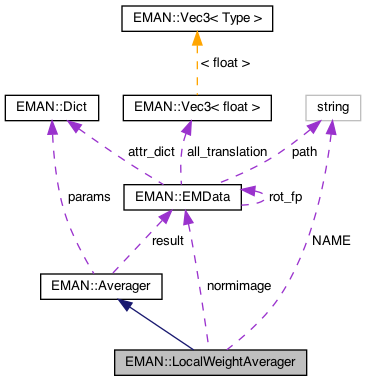EMAN2
EMAN::LocalWeightAverager Class Reference

LocalWeightAverager makes an average of a set of images in Fourier space using a per-image radial weight. More...

`#include <averager.h>`

Inheritance diagram for EMAN::LocalWeightAverager:[legend]
Collaboration diagram for EMAN::LocalWeightAverager:[legend]

## Public Member Functions

LocalWeightAverager ()

To add an image to the Averager. More...

EMDatafinish ()
Finish up the averaging and return the result. More...

string get_name () const
Get the Averager's name. More...

string get_desc () const

TypeDict get_param_types () const
Get Averager parameter information in a dictionary. More...Public Member Functions inherited from EMAN::Averager
Averager ()

virtual ~Averager ()

virtual void add_image_list (const vector< EMData * > &images)
To add multiple images to the Averager. More...

virtual void set_params (const Dict &new_params)
Set the Averager parameters using a key/value dictionary. More...

virtual void mult (const float &s)
Multiply the result image by some floating point constant This is useful when weighting the input images prior to calling add_image - a situation where it is likely you want to divide by the sum of the weights. More...

## Static Public Member Functions

static AveragerNEW ()

## Static Public Attributes

static const string NAME = "localweight"

## Private Attributes

std::vector< EMData * > images

EMDatanormimage

int freenorm

int nimg

int fourierProtected Attributes inherited from EMAN::Averager
Dict params

EMDataresult

## Detailed Description

LocalWeightAverager makes an average of a set of images in Fourier space using a per-image radial weight.

The provided XYData object for each inserted image should range from x=0 - 0.5*sqrt(2), and contains the radial weights from 0 - Nyquist at the point. If the x range is insufficient, values will be clamped at the ends of the available x-range. 2-D Images only, but will work with rectangular images.

Parameters
 normimage After finish() will contain the sum of the weights in each Fourier location. Size must be ((nx+1)/2,y)

Definition at line 214 of file averager.h.

## ◆ LocalWeightAverager()

 LocalWeightAverager::LocalWeightAverager ( )

Definition at line 549 of file averager.cpp.

Referenced by NEW().

## Member Function Documentation

 void LocalWeightAverager::add_image ( EMData * image )
virtual

To add an image to the Averager.

This image will be averaged in this function.

Parameters
 image The image to be averaged.

Reimplemented from EMAN::Averager.

Definition at line 555 of file averager.cpp.

556{
557 if (!image) {
558 return;
559 }
560
561 nimg++;
562
563 int nx = image->get_xsize();
564 int ny = image->get_ysize();
565 int nz = image->get_zsize();
566
567 fourier = params.set_default("fourier", (int)0);
568 if (nimg == 1) {
570 result->set_size(nx, ny, nz);
571 result->to_zero();
572
573
574 normimage = params.set_default("normimage", (EMData*)0);
575 if (normimage==0) { normimage=new EMData(nx,ny,nz); freenorm=1; }
576 normimage->to_zero();
578 }
579
580 images.push_back(image->copy());
581}
EMData * result
Definition: averager.h:158
type set_default(const string &key, type val)
Default setting behavior This can be achieved using a template - d.woolford Jan 2008 (before there wa...
Definition: emobject.h:569
EMData stores an image's data and defines core image processing routines.
Definition: emdata.h:82
std::vector< EMData * > images
Definition: averager.h:250

## ◆ finish()

 EMData * LocalWeightAverager::finish ( )
virtual

Finish up the averaging and return the result.

Returns
The averaged image.

Implements EMAN::Averager.

Definition at line 583 of file averager.cpp.

584{
585 if (nimg==0) return NULL;
586
587 int nx = images.front()->get_xsize();
588 int ny = images.front()->get_ysize();
589 int nz = images.front()->get_zsize();
590
591 float dampnoise = params.set_default("dampnoise", (float)0.5);
592
593 // This is the standard mode where local real-space correlation with the average is used to define a local weight (like a mask)
594 // applied to each image. If fourier>=2 then the same is done, but in "gaussian bands" in Fourier space
595 if (fourier<=1) {
596 EMData *stg1 = new EMData(nx,ny,nz);
597
598 for (std::vector<EMData*>::iterator im = images.begin(); im!=images.end(); ++im) stg1->add(**im);
599 stg1->process_inplace("normalize");
600
601 // std::vector<EMData*> weights;
602 for (std::vector<EMData*>::iterator im = images.begin(); im!=images.end(); ++im) {
603 EMData *imc=(*im)->copy();
604 imc->mult(*stg1);
605 imc->process_inplace("filter.lowpass.gauss",Dict("cutoff_freq",0.02f));
606 imc->process_inplace("threshold.belowtozero",Dict("minval",0.0f));
607 // imc->process_inplace("math.sqrt");
608 // imc->process_inplace("math.pow",Dict("pow",0.25));
609 (*im)->mult(*imc);
612 delete imc;
613 delete *im;
614 }
615
616 if (dampnoise>0) {
617 float mean=normimage->get_attr("mean");
618 float max=normimage->get_attr("maximum");
619 normimage->process_inplace("threshold.clampminmax",Dict("minval",mean*dampnoise,"maxval",max));
620 }
621
622 result->div(*normimage);
623
624 result->set_attr("ptcl_repr",nimg);
625
626 if (freenorm) { delete normimage; normimage=(EMData*)0; }
627 nimg=0;
628
629 return result;
630 }
631 else if (fourier<=ny/2) {
632 // we do pretty much the same thing as above, but one Fourier "band" at a time
633
634 for (int r=0; r<fourier; r++) {
635 float cen=r/((float)fourier-1.0)*0.5;
636 float sig=(0.5/fourier)/(2.0*sqrt(log(2.0)));
637 std::vector<EMData*> filt;
638
639 EMData *stg1 = new EMData(nx,ny,nz);
640 for (std::vector<EMData*>::iterator im = images.begin(); im!=images.end(); ++im) {
641 EMData *f=(*im)->process("filter.bandpass.gauss",Dict("center",(float)cen,"sigma",sig));
642 filt.push_back(f);
644 if (r==fourier-1) delete *im; // we don't actually need the unfiltered images again
645 }
646 stg1->process_inplace("normalize");
647 stg1->process("filter.bandpass.gauss",Dict("center",(float)cen,"sigma",sig));
648// stg1->write_image("cmp.hdf",r);
649
650 // std::vector<EMData*> weights;
651 int imn=1;
652 for (std::vector<EMData*>::iterator im = filt.begin(); im!=filt.end(); ++im) {
653 EMData *imc=(*im)->copy();
654 imc->mult(*stg1);
655 imc->process_inplace("filter.lowpass.gauss",Dict("cutoff_freq",0.02f));
656 imc->process_inplace("threshold.belowtozero",Dict("minval",0.0f));
657// imc->write_image("cmp.hdf",imn*fourier+r);
658 // imc->process_inplace("math.sqrt");
659 // imc->process_inplace("math.pow",Dict("pow",0.25));
660 (*im)->mult(*imc);
663 delete *im;
664 delete imc;
665 imn++;
666 }
667
668 if (dampnoise>0) {
669 float mean=normimage->get_attr("mean");
670 float max=normimage->get_attr("maximum");
671 normimage->process_inplace("threshold.clampminmax",Dict("minval",mean*dampnoise,"maxval",max));
672 }
673
674 }
675 result->div(*normimage);
676 result->set_attr("ptcl_repr",nimg);
677
678 if (freenorm) { delete normimage; normimage=(EMData*)0; }
679 nimg=0;
680 return result;
681 }
682
683}
Dict is a dictionary to store <string, EMObject> pair.
Definition: emobject.h:385
EMData * log() const
return natural logarithm image for a image
EMData * sqrt() const
return square root of current image

## ◆ get_desc()

 string EMAN::LocalWeightAverager::get_desc ( ) const
inlinevirtual

Implements EMAN::Averager.

Definition at line 227 of file averager.h.

228 {
229 return "Average of images weighted by local similarity to unweighted average";
230 }

## ◆ get_name()

 string EMAN::LocalWeightAverager::get_name ( ) const
inlinevirtual

Get the Averager's name.

Each Averager is identified by a unique name.

Returns
The Averager's name.

Implements EMAN::Averager.

Definition at line 222 of file averager.h.

223 {
224 return NAME;
225 }
static const string NAME
Definition: averager.h:247

References NAME.

## ◆ get_param_types()

 TypeDict EMAN::LocalWeightAverager::get_param_types ( ) const
inlinevirtual

Get Averager parameter information in a dictionary.

Each parameter has one record in the dictionary. Each record contains its name, data-type, and description.

Returns
A dictionary containing the parameter info.

Reimplemented from EMAN::Averager.

Definition at line 237 of file averager.h.

238 {
239 TypeDict d;
240// d.put("weight", EMObject::XYDATA, "Radial weight. X: 0 - 0.5*sqrt(2). Y contains weights.");
241 d.put("normimage", EMObject::EMDATA, "After finish() will contain the sum of the weights in real-space");
242 d.put("dampnoise", EMObject::FLOAT, "Will set a minimum mean*x for the norm image, damping regions with poor information. Default = 0.5, 0 disables. ");
243 d.put("fourier", EMObject::INT, "If set does local weighting in Fourier rather than real space ");
244 return d;
245 }

## ◆ NEW()

 static Averager * EMAN::LocalWeightAverager::NEW ( )
inlinestatic

Definition at line 232 of file averager.h.

233 {
234 return new LocalWeightAverager();
235 }

References LocalWeightAverager().

## ◆ fourier

 int EMAN::LocalWeightAverager::fourier
private

Definition at line 254 of file averager.h.

## ◆ freenorm

 int EMAN::LocalWeightAverager::freenorm
private

Definition at line 252 of file averager.h.

## ◆ images

 std::vector EMAN::LocalWeightAverager::images
private

Definition at line 250 of file averager.h.

## ◆ NAME

 const string LocalWeightAverager::NAME = "localweight"
static

Definition at line 247 of file averager.h.

Referenced by get_name().

## ◆ nimg

 int EMAN::LocalWeightAverager::nimg
private

Definition at line 253 of file averager.h.